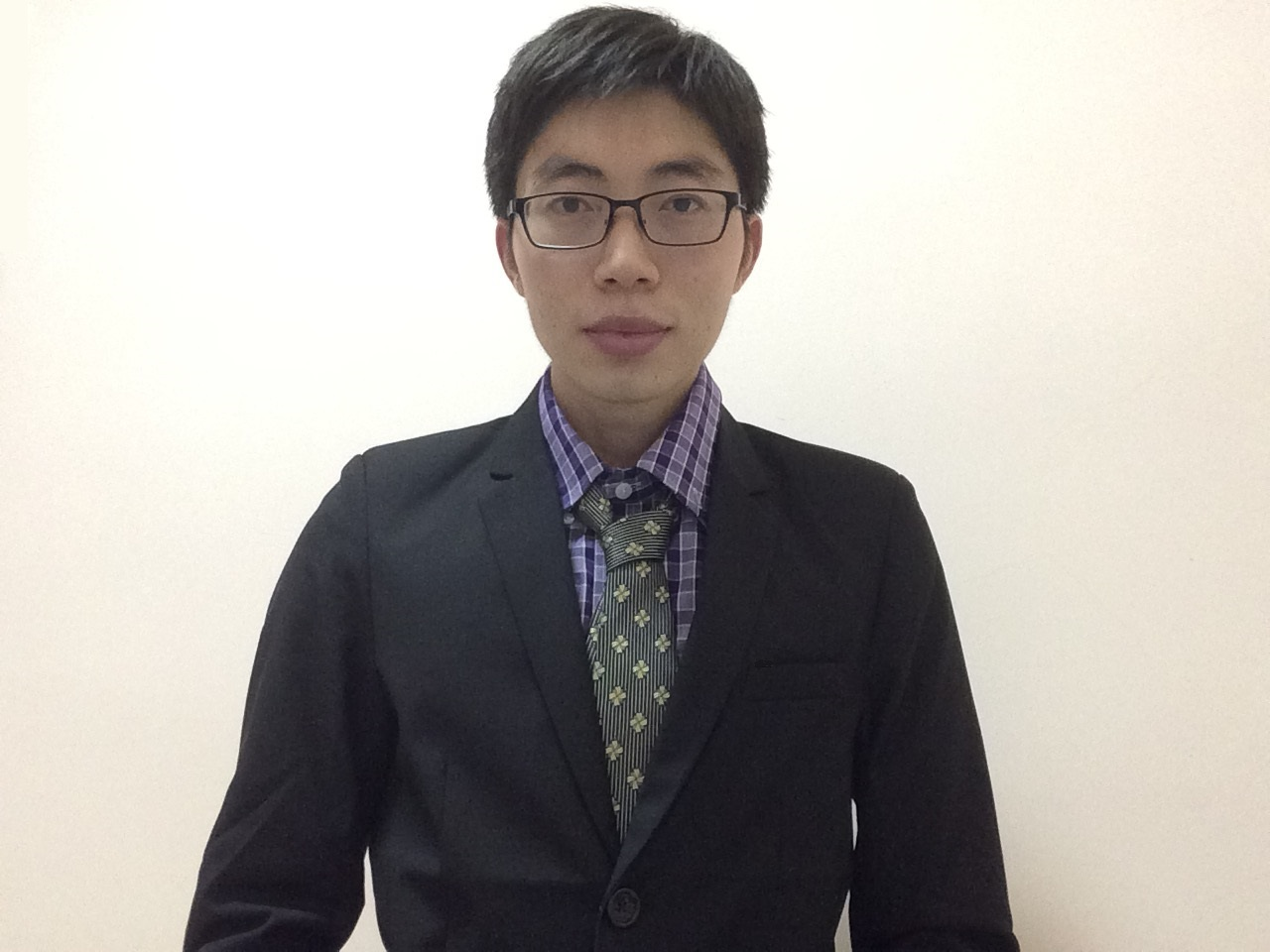## 学术成果 查看更多

Abdelkader Baayoun, Roger E. Khayat* &  Yunpeng Wang. 2022. The transient spread of a circular liquid jet and hydraulic jump formation. Journal of Fluid Mechanics. Accepted.

Yunpeng Wang, Zelong Yuan, Chenyue Xie & Jianchun Wang*. 2022. Temporally sparse data assimilation for the small-scale reconstruction of turbulence. Physics of Fluids. Vol. 34, 065115.

Chao Zhang, Zelong Yuan, Yunpeng Wang, Ruibo Zhang and Jianchun Wang*. 2022. Density-unweighted subgrid-scale models for large-eddy simulations of compressible turbulence. Physics of Fluids. Vol. 34, 065137.

Zelong Yuan, Yunpeng Wang, Chenyue Xie and Jianchun Wang*. 2022. Dynamic nonlinear algebraic models with scale-similarity dynamic procedure for large-eddy simulation of turbulence. Advances in Aerodynamics, Vol. 4, 16.

Yunpeng Wang, Zelong Yuan, Chenyue Xie & Jianchun Wang*. 2021. A dynamic spatial gradient model for the subgrid closure in large-eddy simulation of turbulence. Physics of Fluids. Vol. 33, 075119.

Yunpeng Wang, Zelong Yuan, Chenyue Xie & Jianchun Wang*. 2021. Artificial neural network-based spatial gradient models for large-eddy simulation of turbulence. AIP Advances. Vol. 11, 055216.

Yunpeng Wang*, Roger E. Khayat & Asif Zobaer. 2021. Onset of thermal convection of a weakly rarefied Maxwellian gas: a continuum-slip approach. Physics of Fluids. Vol. 33, 026102.

Yunpeng Wang & Roger E. Khayat*. 2021. The effects of gravity and surface tension on the circular hydraulic jump for low- and high-viscosity liquids: a numerical investigation. Physics of Fluids. Vol. 33, 012105.

Yunpeng Wang & Roger E. Khayat*. 2021. The planar spread of a liquid jet and hydraulic jump on a porous layer. Physics of Fluids. Vol. 33, 012104.

Zelong Yuan, Yunpeng Wang, Chenyue Xie & Jianchun Wang*. 2021. Dynamic iterative approximate deconvolution models for large eddy simulation of turbulence. Physics of Fluids. Vol. 33, 085125.

Zelong Yuan, Yunpeng Wang, Chenyue Xie & Jianchun Wang*. 2021. Deconvolutional artificial-neural-network framework for subfilter-scale models of compressible turbulence. Acta Mechanica Sinica. Vol. 37, 1775-1787.

Yunpeng Wang & Roger E. Khayat*. 2020. The influence of heating on liquid jet spreading and hydraulic jump. Journal of Fluid Mechanics. Vol. 883, A59.

Yunpeng Wang & Roger E. Khayat*. 2020. An asymptotic-numerical comparative study for axisymmetric free-surface liquid jet near and far from exit at high Reynolds number. International Journal of Numerical Methods for Heat and Fluid Flow. Vol. 30, No. 10, 4493-4527.

Yunpeng Wang & Roger E. Khayat*. 2019. The role of gravity in the prediction of the circular hydraulic jump radius for high-viscosity liquids. Journal of Fluid Mechanics. Vol. 862, 128-161.

Yunpeng Wang & Roger E. Khayat*. 2019. A fast two-way coupled integral approach for impinging jet heat transfer. CSME-CFDSC Congress 2019.

Yunpeng Wang & Roger E. Khayat*. 2018. Impinging jet flow and hydraulic jump on a rotating disk. Journal of Fluid Mechanics. Vol. 839, 525-560.

## 团队成员 查看更多

wangyp@sustech.edu.cn